### Home > CALC > Chapter 12 > Lesson 12.2.2 > Problem12-78

12-78.
1. Examine the integrals below. Consider the multiple tools available for integrating and use the best strategy. After evaluating each integral, write a short description of your method. Homework Help ✎

1.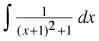2.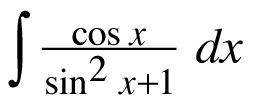3.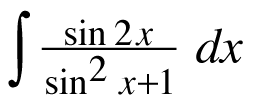4.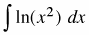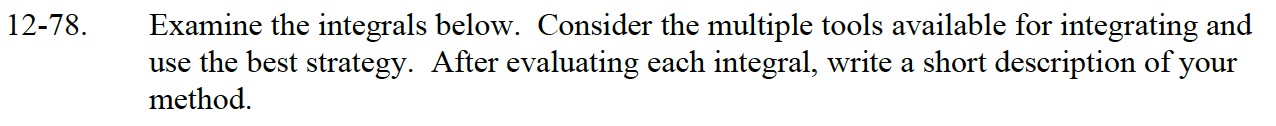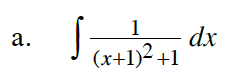Let u = x + 1.
Once you rewrite the integrand, you should recognize it as a special case.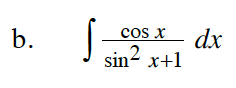Let u = sin(x).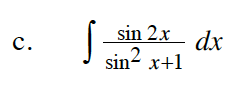sin(2x) = 2sin(x)cos(x)

Let u = sin2(x) + 1.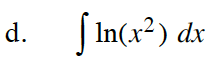ln(x2) = 2ln(x)